HomeBlog

# What Is A Resistor? Basics about Resistors

What is a Resistor?

Resistors are passive electrical components that limit electric current. This video explains in an easy way the most basic background to help you understand resistors and use them.

Catalog

 Article Core Resistor’s Basics Concept Resistor and Resistance Structure Resistor Basics Operation Theory Resistor Electronic Symbols Notation Marking method for Resistance and Tolerance of Resistors Method① Direct Scaling Method② Resistor Color Bands/CodesTolerance Identification of rated Power of Resistors IntroductionLoad CharacteristicResistance Temperature Coefficient Resistor Characteristics Resistors in Series and Parallel Termination and Mounting Resistor Types 1. Classified by Volt-ampere Characteristic2. Classified by Material1) Wirewound Resistors2) Carbon composite Resistors3) Carbon Film Resistors4) Metal Film Resistors5) Metal Oxide Film Resistors Special Resistors 1) KNP-RF2) Sensitive Resistors Resistors Selection 1) Fixed Resistors1) Fuse ResistorsThree Basic Principles Attention Detection 1. Appearance Check2. Multimeter Detection① Detection of Fixed Resistance② Detection of Fuse Resistors and Sensitive Resistors③ Detection of Variable Resistors and Potentiometer3. Measuring Resistance with Bridge Development and Research HistoryDevelopment Direction Ideal Resistor Limitation of Real Resistor Recognition Methods (examples expression) 1. Color bands resistors2. Chip resistors Function

Resistor and Resistance

Resistor is a current limiting element in daily life. Resistance is a physical quantity describing the conductivity of a conductor, represented by R. Resistance is defined by the ratio of the voltage U at the two ends of the conductor and the current I passing through the conductor, that is, R=V/I. When the resistor is connected to the circuit, the resistor's resistance is fixed by two pins, which can limit the current to flow through the branch of the resistor. The resistance that cannot be changed is called a fixed resistor. In addition, a variable resistance is called a potentiometer or a variable resistor. The ideal resistor is linear, that is, the instantaneous current of the resistor is positive to the applied instantaneous voltage, for example, variable-voltage resistor is used as a voltage divider. On a bare resistor, there is one or two removable metal contacts, and the contact position determines the resistance between any end of the resistor and the contact.

The terminal voltage and current have a definite function relation, and the two-terminal device which embodies the conversion of electric energy into other forms, represented by the letter R, and its unit is Ω. Devices such as light bulbs, heating wires, resistors and so on can be expressed as resistor elements.

The resistance value of resistor is generally related to temperature, material, component length, and cross section area. The physical quantity of measuring resistance affected by the temperature is temperature coefficient, which is defined as the percentage of resistance value changing when the temperature rises 1℃. The main physical characteristic of resistors is transforming the electricity into the heat energy, also resistor can be said to be an energy dissipation element, because the internal energy will be generated when current flow through it. Resistors usually act as a divider and shunt in a circuit. And for signals, both AC and DC signals can pass through resistors.

In physics, resistance is a figure to indicate the magnitude of a conductor's hindrance to current. The greater the resistance of a conductor, the greater the resistance of the conductor to the current. Generally, different conductors have different resistances, in other words, resistance is a characteristic of the conductor itself. Resistance element is a kind of energy dissipation element which hinders the current. The stuff under the action of matter called resistive substance because of its blocking effect on the current. The resistor will lead to the change of the electron flux. When the voltage at both ends of the conductor is fixed, the larger the resistance, the smaller the current passing through, on the other hand, the smaller the resistance, the greater the current passing through.

Structure

Resistor refers to a two-terminal electronic element made of resistor material with a certain structure which can limit the current passing through the circuit. It is the most widely used component in electronic circuits, which usually form different series according to its power and resistance values. Its function in circuits is to regulate and stabilize current and voltage, that is,  used as shunt and voltage divider, or circuit matching load. According to the circuit requirements, it can also be used for negative feedback or positive feedback of amplifying circuit, voltage-current conversion, voltage or current protecting element when existing input overload. With capacitor, it can form a RC circuit, which can be used as oscillation, filter, bypass, differential, Integral and time constant elements, etc. Those whose resistance values cannot be changed are called fixed resistors, and those with variable resistance values are called potentiometers or variable resistors. Some special resistors, such as thermistors, varistors and sensitive elements, have a nonlinear relationship between voltage and current.

Resistor Basics

The resistor consists of three parts of a resistor body, a framework and a lead-out end (the resistor body of the solid-core resistor is integrated with the framework), and the resistor body plays a important part in this electronic component. For a resistor body with a uniform cross-section, the resistance value is calculated by the following formula: ρ is the electrical resistivity (ohm cm), L is the length (cm) of the resistor, and A is the cross-sectional area of the resistor (square centimeter).

R=ρ× L/A

The thickness of the thin film resistor is very small and difficult to measure accurately, and ρ varies with the thickness values, so the constant related to the film material is regarded as the film resistance. In fact, it is the resistance of the square film, so it is also known as the square resistance. For homogeneous films, W is the width of the film (cm), in general, Rs should be in a limited range, because large Rs will affect the stability of resistor performance. Therefore the cylindrical resistor is notched and the planar resistor uses etching method of detour to extend the range of resistance and to fine-tune the resistance.

R=Rs×L/W

The volt-ampere characteristic is a graph that represents the relationship between the voltage and the current of the resistor terminal. When the voltage-current is proportional (representing a straight line), it is called a linear resistor, otherwise referred to as a non-linear resistor.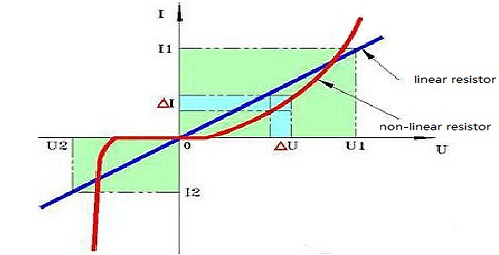The vertical coordinates of this diagram are current I, and the transverse coordinates are voltage U. This graph is also called the current-voltage curve, more often it referred to as the volt-ampere characteristics curve. As we can see, for the blue curve, it is a straight line passing through the origin, and its resistance does not change with the change of voltage and current, in fact, elements that satisfy this volt-ampere characteristic curve are called linear elements. On the other hand, for the red curve, we can see that it is a curve, its resistance value changes with the change of voltage and current.

The main parameters used to characterize the resistance have nominal resistance, allowable deviation, rated power, load characteristic, resistor temperature coefficient, and so on.

Operation Theory

Ohm's law

The behaviour of an ideal resistor is dictated by the relationship specified by Ohm's law:

V=I ∙ R

Ohm's law states that the voltage (V) across a resistor is proportional to the current (I), where the constant of proportionality is the resistance (R). For example, if a 300 ohm resistor is attached across the terminals of a 12 volt battery, then a current of 12/300 = 0.04 amperes flows through that resistor.

Practical resistors also have some inductance and capacitance which affect the relation between voltage and current in alternating current circuits.

In addition, linear or ohmic resistance meets the Ohm's law, but nonlinear resistance does not satisfy the Ohm's law.

The nominal resistance is the design resistance of the sign on the resistor with a digital or color code. The units are Ω, KΩ and MΩ. Resistance values are written according to standardized priority series, which correspond to allowable deviations.

The electrical resistance of a resistor is measured in ohms. The symbol for an ohm is the greek capital-omega: Ω. The ohm (symbol: Ω) is the SI unit of electrical resistance, named after Georg Simon Ohm. An ohm is equivalent to a volt per ampere. Since resistors are specified and manufactured over a very large range of values, the derived units of milliohm (1 mΩ = 10−3 Ω), kilohm (1 kΩ = 103 Ω), and megohm (1 MΩ = 106 Ω) are also in common usage. For example, a 4,700Ω resistor is equivalent to a 4.7kΩ resistor, and a 5,600,000Ω resistor can be written as 5,600kΩ or (more commonly as) 5.6MΩ.

Resistor Electronic symbolsNotation

1Ω= 1 Ohms

1 KΩ= 1 Kilo Ohms

1 MΩ= 1 Mega Ohms

1 GΩ= 1 Giga Ohms

When the value can be expressed without a prefix (that is, multiplicator 1), an "R" is used instead of the decimal separator. For example, 1R3 indicates 1.3Ω, and 15R indicates 15Ω.

Marking method for Resistance and Tolerance of Resistors

① Direct Scaling Method

The resistance and error of the resistor are directly printed on the resistor by numbers and letters (no error is indicated as the tolerance ±20%). There are also manufacturers who use customary marking methods, such as:

3Ω3Ⅰis represent the resistance 3.3Ω, tolerance is ±5%

1 K 8 is represent the resistance 1.8 KΩ, tolerance is ±20%

5 M 1Ⅱis represent the resistance 5.1 MΩ, tolerance is ±10%

② Resistor Color Bands/Codes

This Physics video tutorial is explaining the fundamentals of color code for four colour bands in a carbon resistor.

The color bands are coated on the resistor to indicate the nominal value of the resistor and the allowable tolerance. The corresponding values of various colors and the recognition rules of the color bands/codes of reading principle of the fixed resistor are shown in the following figure.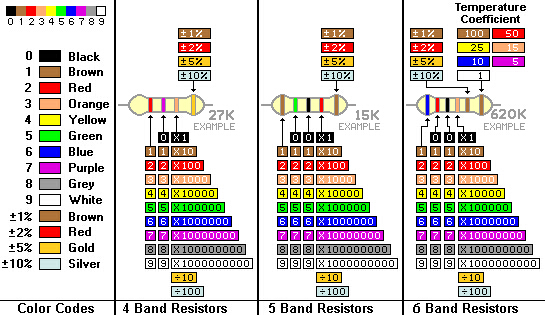Four-band Resistors

In the standard four band resistors, the first two bands indicate the two most-significant digits of the resistor’s value. The third band is a weight value, which multiplies the two significant digits by a power of ten.

The final band indicates the tolerance of the resistor. The tolerance explains how much more or less the actual resistance of the resistor can be compared to what its nominal value is. No resistor is made to perfection, and different manufacturing processes will result in better or worse tolerances. For example, a 1kΩ resistor with 5% tolerance could actually be anywhere between 0.95kΩ and 1.05kΩ.

Example: red orange black gold=23*10^0=23Ω(±5%)

Five-band and six-band Resistors

Five band resistors have a third significant digit band between the first two bands and the multiplier band. Five band resistors also have a wider range of tolerances available.

Six band resistors are basically five band resistors with an additional band at the end that indicates the temperature coefficient. This indicates the expected change in resistor value as the temperature changes in degrees Celsius. Generally these temperature coefficient values are extremely small, in the ppm range.

Example: red blue green black brown=265*10^0=265Ω(±1%)

Tolerance

The maximum tolerance between the actual resistance and the nominal resistance, represented by percentages. Commonly used are ±5%, ±10%, ±20%, precision is less than ±1%, high precision up to 0.001%. The accuracy is determined by both the tolerance and the irreversible resistance.

Power Rating

Maximum power dissipation allowed for continuous operation of resistors at rated temperature (maximum ambient temperature) tR. And the maximum operating voltage is also specified for each resistor, that is, the maximum operating voltage cannot be exceeded even if the resistance value is high.

Identification of rated Power of Resistors

The rated power of a resistor refers to the maximum power that a resistor is allowed to consume in a long-term continuous operation in a DC or AC circuit. There are two marking methods: the resistance above 2W is directly printed on the resistor body, and the resistance below 2W is represented by its own volume. When the resistance power is expressed on the circuit diagram, the following symbols are used: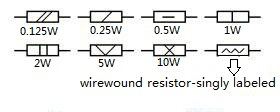1) Load Characteristic

When the working temperature is lower than tR, the resistor can not exceed its rated power, and the load power must be reduced when it exceeds tR. Each resistor has its own specified load characteristic. In addition, the load is allowed to be reduced accordingly at low pressure. Under pulse load, the average power of pulse is much lower than the rated power, the load is according to the practical situation.

2) Resistance Temperature Coefficient

The average relative variation of the resistance value at each change of 1℃ is expressed in terms of ppm/ ℃ within a specified range of ambient temperature. In addition to the above parameters, there are other technical indicators, such as nonlinearity (the extent to which the characteristics of the current and the applied voltage deviate from the linear relationship), the voltage coefficient (the relative rate of change of the voltage at which the voltage is applied, the relative rate of change of the volt resistance), current noise (the ratio of the effective value of the noise potential generated by the current flow in the resistor to the measured voltage, expressed by the current noise index), the high frequency characteristic (due to the effect of the distributed capacitance and the distributed inductance in the resistor; a curve in which the resistance value decreases as the operating frequency increases; long-term stability (such as irreversible changes in resistance values affected by environmental conditions during long term use or storage).

Resistor Characteristics

Dependent on the application, the electrical engineer specifies different properties of the resistor. The primary purpose is to limit the flow of electrical current; therefore the key parameter is the resistance value. The manufacturing accuracy of this value is indicated with the resistor tolerance in percentage. Many other parameters that affect the resistance value can be specified, such as long term stability or the temperature coefficient. The temperature coefficient, usually specified in high precision applications, is determined by the resistive material as well as the mechanical design.

In high frequency circuits, such as in radio electronics, the capacitance and inductance can lead to undesired effects. Foil resistors generally have a low parasitic reactance, while wirewound resistors are amongst the worst. For accurate applications such as audio amplifiers, the electric noise must be as low as possible. This is often specified as microvolts noise per volt of applied voltage, for a 1 MHz bandwidth. For high power applications the power rating is important. This specifies the maximum operating power the component can handle without altering the properties or damage. The power rating is usually specified in free air at room temperature. Higher power ratings require a larger size and may even require heat sinks. Many other characteristics can play a role in the design specification. Examples are the maximum voltage, or the pulse stability. In situations where high voltage surges could occur this is an important characteristic.

Resistors in series and parallel Connection

In electronic circuits, resistors are very often connected in series or in parallel. A circuit designer might for example combine several resistors with standard values (E-series) to reach a specific resistance value. For series connection, the current through each resistor is the same and the equivalent resistance is equal to the sum of the individual resistors. For parallel connection, the voltage through each resistor is the same, and the inverse of the equivalent resistor value is equal to the sum of the inverse values for all parallel resistors. In the articles resistors in parallel and series a detailed description of calculation examples is given. To solve even more complex networks, Kirchhoff’s circuit laws may be used.

The total resistance of resistors connected in series is the sum of its each single individual resistance value.The total resistance of resistors connected in parallel is the reciprocal of the sum of the reciprocals of the individual resistors.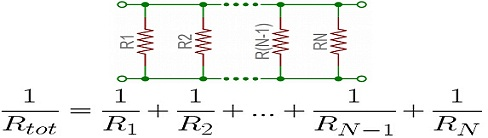As a special case of this equation: if you have just two resistors in parallel, their total resistance can be calculated with this slightly-less-inverted equation: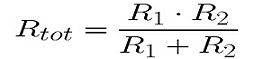Termination and Mounting

Resistors will come in one of two termination-types: through-hole or surface-mount. These types of resistors are usually abbreviated as either PTH (plated through-hole) or SMD/SMT (surface-mount technology or device).

Through-hole resistors come with long, pliable leads which can be stuck into a breadboard or hand-soldered into a prototyping board or printed circuit board (PCB). These resistors are usually more useful in breadboarding, prototyping, or in any case where you’d rather not solder tiny, little 0.6mm-long SMD resistors. The long leads usually require trimming, and these resistors are bound to take up much more space than their surface-mount counterparts.

The most common through-hole resistors come in an axial package. The size of an axial resistor is relative to its power rating. A common 1/2W resistor measures about 9.2mm across, while a smaller 1/4W resistor is about 6.3mm long.

Surface-mount resistors are usually tiny black rectangles, terminated on either side with even smaller, shiny, silver, conductive edges. These resistors are intended to sit on top of PCBs, where they’re soldered onto mating landing pads. Because these resistors are so small, they’re usually set into place by a robot, and sent through an oven where solder melts and holds them in place.

Resistor Types

Most types of resistor are linear devices that produce a voltage drop across themselves when an electrical current flows through them because they obey Ohm’s Law, and different values of resistance produces different values of current or voltage. This can be very useful in Electronic circuits by controlling or reducing either the current flow or voltage produced across them we can produce a voltage-to-current and current-to-voltage converter.

Resistors come in a variety of shapes and sizes. They might be through-hole or surface-mount. They might be a standard, static resistor, a pack of resistors, or a special variable resistor. The different types of resistor are discussed in the following section.

1. Classified by Volt-ampere Characteristic

*Linear resistors

*Non-linear resistorsFor most conductors, at a certain temperature, the resistance is almost unchanged and is a certain value, the resistors having this kind of resistance is called linear resistor. The resistors of some materials varies obviously with the current (or voltage) change, and the volt-ampere characteristic of them is a curve, which is called nonlinear resistor. Under a given voltage (or current), the ratio of voltage to current is the static resistance at the working point, and the slope on the voltage-ampere characteristic curve is dynamic resistance. The expression of nonlinear resistance characteristic is complicated, but these nonlinear relations are widely used in electronic circuits.

2. Classified by Material

1) Wirewound resistor is made of resistive wires, wound high resistance alloy wires on an insulating skeleton, and coated with a heat-resistant glaze insulating layer or insulating paint. The wirewound resistor has low temperature coefficient, high resistance accuracy, good stability, sound heat resistance and corrosion resistance. It is mainly used as precise and high power resistance. The shortcoming is that the high-frequency performance is poor and the time constant is large.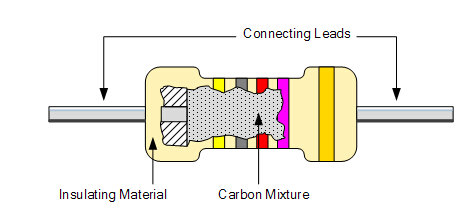Applicationss

·It has high security

·Accurate measurement and balance current control is required.

2) The carbon composition resistor is made from the mixer of granulated or graphite, insulation filter and a resin binder. The actual resistance of the resistor is determined by the ratio of the insulation material. The shape of the insulating binder is in the shape of roads and there are two metal caps at both the end of the roads. At both ends of the resistor it has two wire conductors for easy to connectivity in the circuit design. There are different colors which are printed on the resistor to find the value of it and the road is covered with the plastic coat.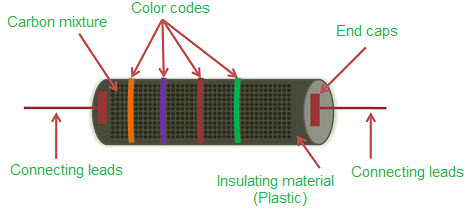Applications

The composition resistor is used in the high energy pulses.

·It has a relatively small size.

·High voltage power supplies

·Welding·High power

3) A carbon film resistor is plated with a carbon layer on the porcelain tube, and the crystalline carbon is deposited on the ceramic rod framework. Further more, the temperature coefficient is from the -100 to -900 ppm/°C. The carbon film resistor has the advantages of low cost, stable performance, wide resistance range, low temperature coefficient and low voltage coefficient, and is the most widely used resistor.Applications

·The carbon film resistors are available in High plus stability.

4) A metal film resistor is coated with a layer of metal on the ceramic tube, and the alloy material is plated on the surface of the ceramic rod skeleton by vacuum evaporation.

Metal film resistor is more accurate than carbon film resistor, other advantages such as good stability, small noise, low temperature coefficient. It is widely used in instrumentation and communication equipment.

5) Metal oxide film resistors are coated with tin oxide on the ceramic tube, and a layer of metal oxide is deposited on the insulating rod. Because its body is an oxide, so it has high temperature stability, heat shock resistance, sound load capacity. According to the purpose, it is divided into universal, precision, high frequency, high voltage, high resistance, and high power type, also it can forms resistors network.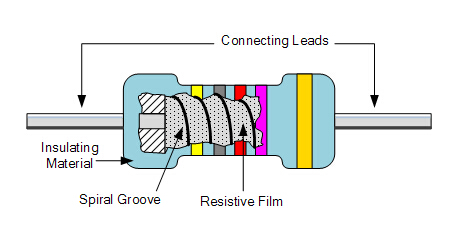Special Resistors

1) KNP-RF: it also called a fuse resistor, which functions as a resistor and a fuse in the normal condition. When the circuit fails to make the power exceed the rated power, it will be blown as if the fuse is blown and the connection circuit is disconnected. The general resistance of the fuse resistance is small (0.33Ω ~ 10KΩ), and the power is also small. The common types of fuse resistors are: RF10 type, RF111-5 fuse resistor symbol type, RRD0910 type, RRD0911 type, etc.

2) Sensitive resistor: a resistor whose resistance value is sensitive to certain physical quantities (such as temperature, humidity, light, voltage, mechanical force, gas concentration, etc.). When these quantities change, The resistance value of the sensitive resistors will change with the change of physical quantity, showing different resistance values. According to the sensitivity to different physical quantities, the sensitive resistor can be classified as heat sensitive, humidity sensitive, photosensitive, pressure-sensitive, force sensitive, magnetic sensitive and gas sensitive. Sensitive resistors are almost made of semiconductor materials, thus they are also known as semiconductor resistors.

The resistance of the thermistor varies with the change of temperature, when the temperature rise, this resistor is a negative temperature coefficient (NTC) thermistor. In most case, the NTC thermistor is widely used, according to its different use, it can be divided into common NTC thermistor, steady-voltage NTC thermistor, thermometric NTC thermistor and so on.

The resistance of the photosensitive resistor changes with the intensity of the incident light. When the incident light is enhanced, the resistance decreases and the resistance increases when the incident light weakens.

Selection

1) There are many types of fixed resistors, what materials and structures should be selected, it is necessary to consider the specific requirements of the application circuit. In high-frequency circuits, non-wirewound resistors with small distributed inductance and capacitance should be selected,  such as carbon film resistor, metal resistor and metal oxide film resistor, thin film resistor, thick film resistor, alloy resistor, corrosion-resistant film resistor, etc. In high gain and small signal amplifying circuits, low noise resistors should be used, such as metal film resistors, carbon film resistors and wirewound resistors, rather than synthetic carbon film resistors and organic solid resistors with high noise.

There are different types of the resistor which are in the following:

Carbon composition

Carbon Pile

Carbon film

Printed carbon resistor

Thick and thin film

Metal film

Metal oxide film

Wire wound

Foil resistor

Ammeter shunt

Grid resistor

Special verities

Led Arrangement

The resistance value of the selected resistor shall be close to a nominal value of the calculated value in the application circuit, and the standard series resistor shall be preferred. The tolerance of resistors used in general circuits is ±5%~±10%. Precision resistors should be used in precision instruments and special circuits, with a precision within 1%, such as 0. 01%, 0.1% or 0. 5% tolerance. The rated power of the selected resistor should not be arbitrarily increased or reduced in order to meet the requirements of the power capacity of the resistor in the application circuits.

If the circuit is required to be a power resistor, the rated power can be 2 times higher than that required by the practical application circuit.

Selection of Fuse Resistor

Fuse resistor, a kind of resistors with protective function. The dual performance should be considered and the parameters such as resistance and power should be selected according to the specific requirements of the circuits. It is not only to ensure that it can fuse quickly when it is overloaded, but also to guarantee that it can work stably for a long time under normal conditions. In addition, if the resistance is too high or the power is too large, it can not play a protective role either.

Three basic principles for the selection of resistors:

Select high-level standard resistors manufactured by a production line certified by the certification administrations.

Select resistors manufactured by manufacturers with advantages of function, quality, efficiency,  price and service.

Select the manufacturer who meets the above requirements.

Attention

The resistors should be checked before use, checking its performance is to measure whether the actual resistance value is consistent with the nominal value and whether the error is within the allowable range. The method is to measure the resistance by the multimeter.

Two points need to pay attention to when measuring.

1)The range should be determined according to the measured resistance, when the pointer is indicated in the middle of the scale, which is easy to observe.

2)After determining the resistor range, having zero adjustment is that the two table pens are directly touching (short circuit), that is, the "zero adjustment" device is adjusted so that the pointer is accurately pointed to the "0" of the Ω scale, and then the resistance value is measured again. Also, be careful not to touch both ends of the resistor or the metal part of the pen, otherwise, the test error will be caused.

If the resistance measured by the multimeter is close to the nominal value, the basic quality is good, and if the difference is big or the multimeter does not work at all, this resistor is bad.

Detection

1. Appearance Check

For fixed resistor, check the logo clear firstly: intact protective paint, no charring, no scars, no cracks, no corrosion, resistive body and pin connected closely. For potentiometers, the axis flexible, proper tight, comfortable handle should be the key points. If there is a switch, checking the switch whether it is working properly.

2. Multimeter Detection

① detection of fixed resistance

When measuring, the different resistances are measured by the proper electric gears of the multimeter. For the pointer type multimeter, because the indicator of electric gear is nonlinear, the larger the resistance value, the more dense the indicator number is, so the more accurate the reading is, the larger the angle of needle deviation should be, to make more accurate reading numbers. If the measured result exceeds the error-range of the resistor, the resistance value is infinite, unstable or the zero, which indicates that the resistor has been broken.

In the measuring process, the hands holding the resistor should not contact with the two pins of the resistor, which will affect the accuracy of the measurement. In addition, the multimeter can not be used to detect the resistance during power on, because on-line detection shouldn’t be allowed.

② Detection of fuse resistors and sensitive resistors

The resistance range of fuse resistors is generally only a few to dozens of Ω. If the detecting resistance is infinite, it indicates that the fuse has been fused. The resistance can also be measured on line, measuring the grounding voltage at both ends respectively, if one end is equal to the power supply voltage and another end voltage is 0V, indicating the fuse resistor has broken.

There are many kinds of sensitive resistors, in this section, taking thermistors as an example. As above mentioned, it is divided into positive temperature coefficient(PTC) thermistors and negative temperature coefficient(NTC) thermistors. For the PTC thermistor, the resistance value is usually small at normal temperature. In the measurement, when using the electric soldering iron with burning heat close to the resistor, the resistance value will be obviously increased, which indicates the resistor is normal, and if no change appears, indicating the component is damaged. The NTC thermistor is the opposite.

Under the circumstance without light, the resistance value of light sensitive resistors is large, on the contrary, the measuring resistance will be reduced obviously when there is light, if there is no change, the component is damaged.

③ Detection of variable resistors and potentiometer

Firstly, measuring whether the resistance values between the two fixed ends is normal, if the detecting values are infinite or zero, or is larger or small than the nominal values, exceeding the tolerances, which indicating the device is damaged. If the resistance value of the resistive body is normal, then a meter pen of the multimeter is connected with the sliding end of the potentiometer, the other pen is connected with any fixed end of the potentiometer (adjustable resistor), and the footstalk is slowly rotated to observe whether the meter needle is stably changed: when the resistance value is changed from zero to the nominal value (or vice versa) from one end to the other end, and there is no jump or jitter in the course of rotation, which indicating the potentiometer is normal, if any, it is indicated that the sliding point of resistance is in poor contact.

3. Measuring resistance with bridge

If accurate measurement of resistor resistance is required, it can be measured by bridge (digital). The resistance can be read from the display by inserting the resistor into the measuring end of the bridge by selecting an appropriate range. For example, when a resistor is made from a wire or a fixed resistor is processed to obtain a more accurate resistance value, under this situation, the resistance of the self-made resistor must be measured by a bridge.

Different applications, the purpose of applying varistor, different voltage/current stress acting on the varistor, so the requirements for the varistors are different, thus it is necessary to pay attention to detecting. According to the purpose of application, the varistors can be divided into two categories: the protection varistor and the circuit function varistor.

1) Distinguish between power supply, signal lines, and data wire protection for varistors, because they should meet the requirements of different technical standards.

2) According to the difference of the continuous working voltage applied on the varistors, the power lines can be divided into two types: AC or DC, in addition, the aging characteristics of varistors under these two voltage stresses are different.

3) According to the abnormal overvoltage of varistors, it can be divided into three types: surge suppression type, high power type and high energy type.

Surge suppression type: it is used to suppress transient overvoltages caused by lightning and improper operation. Transient overvoltages are random, aperiodic, and the peak value of current voltages may be very large.

High power type: it is used to absorb a continuous group of pulses occurring in a period, for example, a varistor connected to a switching power converter, where the impulse voltage period occurs, and the period is knowable, so the energy value can generally be calculated. From this, we can see the peak value of voltage is not large, but occurs frequently, thus its average power is quite large.

High energy type: it is used to absorb magnetic energy in large inductance coils such as generator excitation coils, lifting electromagnet coils, etc. For such applying requirement, the main technical index is energy absorption capacity.

The protection of varistor can be used repeatedly in most applications, but sometimes it can be made into a one-off protection device such as current fuse. For example, a varistor with short-circuit contact connected to a load of some current transformers.

Development and Research

History

In 1885, British C ∙ Bradley invented molded carbon solid core resistor. In 1897, British T ∙ Gambril and A ∙ Harris made carbon film resistor from carbon ink. In 1913 ~ 1919, British W ∙ Swan and Germany F∙ Krueger invented metal film resistor successively. In 1925, Siemens-Halsk company invented thermal decomposition carbon film resistor, which broke the marketing monopoly of carbon solid core resistor. Since the advent of transistors, the miniaturization and the stability of resistors have been required more strictly, which has promoted the development of new types of resistors. In 1959, Bell Labs developed TaN resistor. Since the 1960s, some products have been developed to planarization, integration, miniaturization when using new technologies such as drum magnetron sputtering and laser resistance trimming.

Development Direction

1. Miniaturization, high reliability.

2. Discrete small resistors still have a wide range of applications, but it need further improvement, such as smaller size, higher performance and lower prices.

3. In consumer electronics, carbon film resistor is still in domination, and metal film resistor still take over the precise devices market, in addition, most of small power wirewound resistors will be replaced.

4.In order to meet the development of circuit integration and planarization, the demand for chip resistors will increase obviously, and the general type will tend to develop thick film resistors, while the precision type will still be inclined to metal film and metal foil resistors in film type.

5. Develop the combined resistors network.

Ideal Resistor

In an ideal resistor, the resistance does not change with the voltage or current, nor with the sudden change of the current, but in reality, resistors can't do this. Today's internal design enables resistors to exhibit relatively small resistive variations at extreme voltages or currents (and even other abnormal factors such as temperature).

Limitation of Real Resistor

Each resistor has its upper limit of voltage or current (depending on the size of the resistor). If the voltage or current exceeds this range, the resistance value changes (some may vary dramatically in some resistors), even worse the resistor is damaged by overheating. Most resistors will indicate rated electrical power, others will provide the rated current or voltage. In fact, a real resistor also has a small amount of inductance or capacitance, which makes it different from the ideal resistor.

Recognition Methods

1. Color bands resistor

The first and second band with four color bands represent the first two digits of the resistance respectively; the third band represents the multiplier; the fourth band represents the tolerance.

 Color Digit Multiplier Tolerance Black 0 100 Brown 1 101 1 Red 2 102 2 Orange 3 103 Yellow 4 104 Green 5 105 0.5 Blue 6 106 0.25 Violet 7 107 1 Grey 8 108 White 9 109 Gold 10-1 5 Silver 10-2 10 None 20

(1) The key to fast recognition is to determine the resistance value within a certain magnitude according to the color of the third band, and then to recognize the resistance of first and second band, following this way, the number can be read out quickly.

 Gold units Black tens Brown hundreds Red thousands Orange ten thousands Yellow hundred thousands Green millions Blue ten millions

(2) Memorize the number of colors represented by the first and second rings,the following table is the number of each color represents.

On the order of magnitude, they can be divided into three grades: gold, black, brown is Ω; red, orange, yellow is KΩ; green, blue is MΩ.

(3) When the second band is black, the third band is represented by an intege, and a special case at the time of reading needs to be noted, for example, the third band is red, the resistance value is a whole number kΩ.

(4) Remember the errors represented by the fourth band color, that is, gold is 5%, silver is 10%, colorless is 20%.

Examples

Example 1

When the four color bands are yellow, orange, red and gold successively, because the third ring is red, the resistance range is several kΩ, according to the representing number of yellow and orange is "4" and "3" respectively, the reading number is 4.3 kΩ, and the fourth band is a gold, so the error is 5%.

Example 2

When the four color bands are brown, black, orange and gold in turn, because the third band is orange and the second band is black, the resistance range should be tens of kΩ, the brown is representing “1”, so the reading number is 10 kΩ, the fourth band is gold, its error is 5%.

2. Chip resistors

Chip resistors have the advantages of small size, light weight, high installation density, high seismic resistance, sound anti-interference ability and good high-frequency characteristic. It is widely used in computers, mobile phones, electronic dictionaries, medical e-products, video cameras, VCD machine etc.

Chip components can be divided into three types according to their shape: rectangular, cylindrical and special-shaped. There are resistors, capacitors, inductors, transistors and small integrated circuits. The nominal method of the chip components is different from that of the common components. The following is mainly about the nominal method of chip resistors.

The resistance value of chip resistor is the same as that of a general resistor. There are three methods of nominal resistance, but it is not exactly the same as that of general resistors.

1) Digital nominal method (usually for general rectangular chip resistors)

This method is using a three-digit number on the resistance body to indicate its resistance. Its first digit and second digit are significant digits, and the third digit represents the numbers of "0" added after the significant number, and this digit place does not appear a letter.

Example: “472′” represents “4700Ω”, “151”represents “150”.

If it is a decimal, use "R" to denote "decimal point" and take up an effective digit, and the remaining two digits are valid numbers.

Example: “2R4” represents “2.4Ω”, “R15”represents “0.15Ω”.

2) Colour bands nominal method (used in general cylindrical fixed resistors)

The chip resistor, like general resistors, is usually indicated by four/five/six bands. The first band and second band are significant numbers and the third band is a multiplier.

Function

Small power resistors are usually made of carbon film packaged in plastic shells, while high power resistors are usually wirewound resistors, which are made by metal wires with high resistance wrapped on the porcelain core.

If the resistance value of a resistor is close to 0Ω, the resistor has not any effect on the current. In parallel with this kind of resistors, the circuit will be short-circuited and the current is infinitely large. If a resistance is very large or infinite, the circuit is in series with such a resistor can be regarded as an open circuit, that is, current is 0A. The commonly used resistor in industry is between two these extreme cases. In other words, it has a certain resistance and can pass through a certain current, but the current is not as large as in short circuit. The resistor's current limiting effect is similar to that of a small diameter tube connected to two large diameter tubes to limit water flow. It is defined as the resistance corresponding to that 1V voltage is added to the conductor to generate 1A current, in fact, the term "resistance" refers to a property, whereas in electronic products, it often refers to a component such as a resistor.

You May Also Like

How to Distinguish Resistor Color Codes? (Axial Resistors)

Ref.

KY83-RL3720WT-R033-F

KY83-RCL06121K50FKEA

KY83-P8212

### Related PostsHOW TO BUY ELECTRONIC COMPONENTS
How to buy
Search
Inquiry
Order
Track

Delivery
FedEx
UPS
DHL
TNT

Payment Terms
By PayPal
By Credit Card
By Wire Transfer
By Western Union

After-sales Service
Quality Control
Guarantee
Return & Replacement

About us
Company Profile
Our History
Corporate Culture
Contact us
Join us
© 2008-2018 kynix.com all rights reserved.
Tel:00852-81928838    Email:info@kynix.com